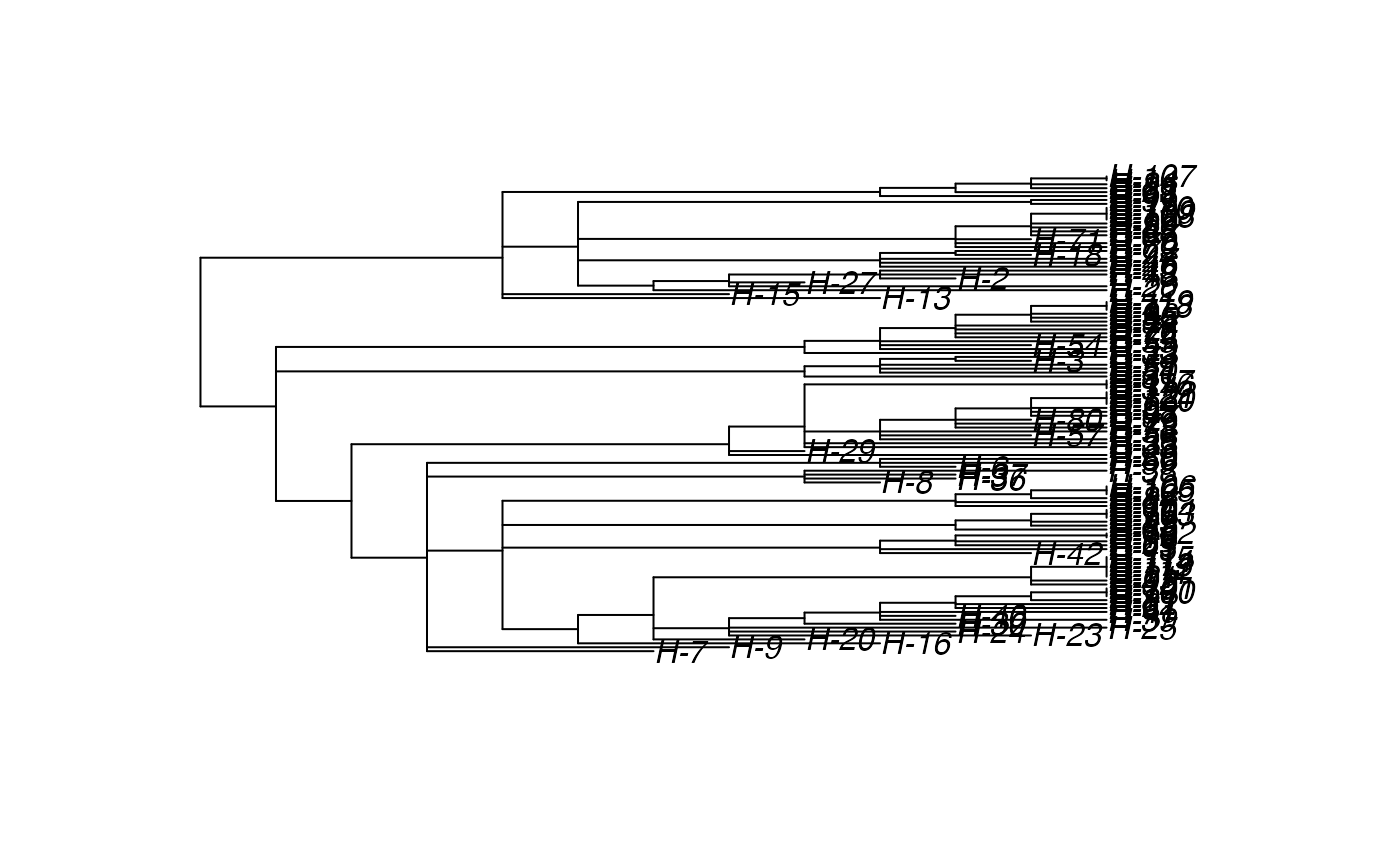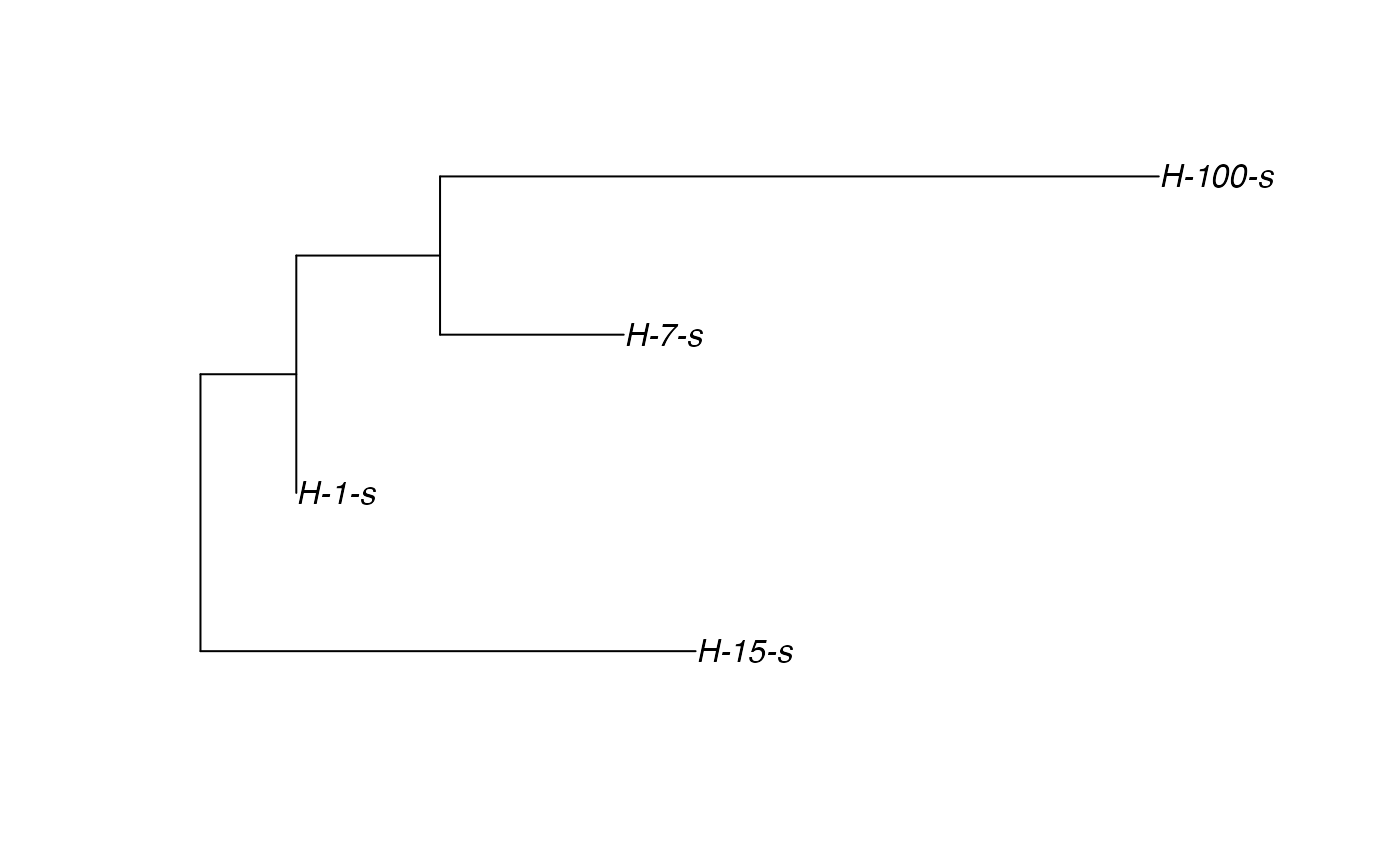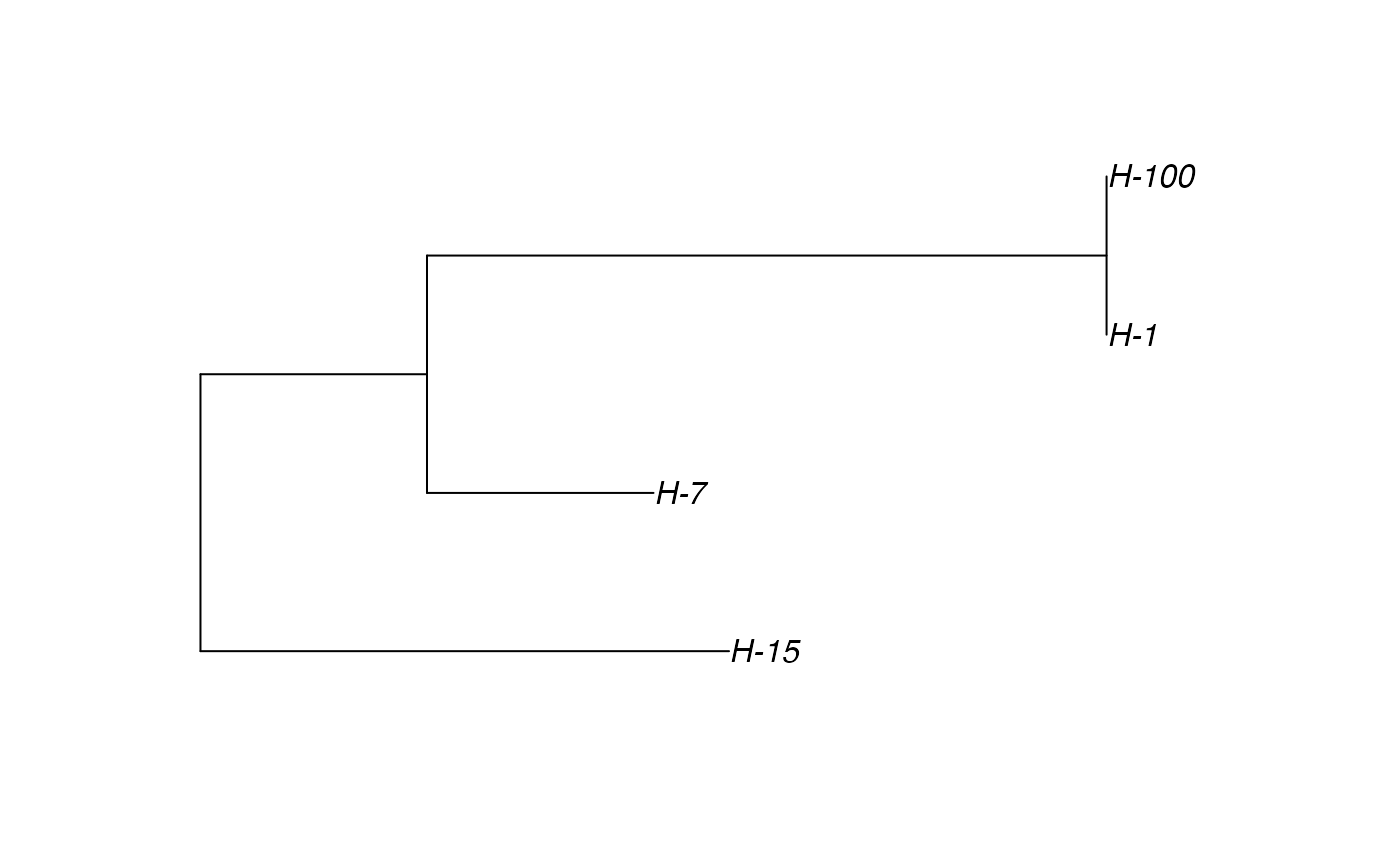Sample a full transmission tree. This function allows for sampling only exited (i.e. inactive) individuals (e.g. when the sampling procedure is destructive or cuts the hosts from the population). Beware because it does not influence the epidemiological process, it only means that the host has been sampled when exiting the simulation.

sampleTransmissionTreeFromExiting(tree, hosts)

## Arguments

tree a treedata object created by function getTransmissionTree a vector of dead hosts to sample

## Value

A tree of class treedata, containing a phylogenetic tree based on the transmission chain and the mapped data at all the nodes.

## Details

The tree needs to be produced by function getTransmissionTree applied on the same nosoiSim object.

For exporting the annotated tree to other software packages, see functions in treeio (e.g. write.beast).

To get the full transmission matrix, see getTransmissionTree.

For sampling among non-dead individuals, see sampleTransmissionTree.

## Examples

# \donttest{
t_incub_fct <- function(x){rnorm(x,mean = 5,sd=1)}
p_max_fct <- function(x){rbeta(x,shape1 = 5,shape2=2)}
p_Exit_fct  <- function(t){return(0.08)}
p_Move_fct  <- function(t){return(0.1)}

proba <- function(t,p_max,t_incub){
if(t <= t_incub){p=0}
if(t >= t_incub){p=p_max}
return(p)
}

time_contact = function(t){round(rnorm(1, 3, 1), 0)}

transition.matrix = matrix(c(0, 0.2, 0.4, 0.5, 0, 0.6, 0.5, 0.8, 0),
nrow = 3, ncol = 3,
dimnames = list(c("A", "B", "C"), c("A", "B", "C")))

set.seed(805)
test.nosoi <- nosoiSim(type="single", popStructure="discrete",
length=20,
max.infected=100,
init.individuals=1,
init.structure="A",
structure.matrix=transition.matrix,
pMove=p_Move_fct,
param.pMove=NA,
nContact=time_contact,
param.nContact=NA,
pTrans = proba,
param.pTrans = list(p_max=p_max_fct,
t_incub=t_incub_fct),
pExit=p_Exit_fct,
param.pExit=NA
)#> Starting the simulation
#> Initializing ...#>  running ...#> Time: 10 (50% of maximum length). Hosts count: 22 (22% of maximum infected hosts).#> done.
#> The simulation has run for 16 units of time and a total of 122 hosts have been infected.
## Make sure all needed packages are here
if (requireNamespace("ape", quietly = TRUE)
|| requireNamespace("tidytree", quietly = TRUE)
|| requireNamespace("treeio", quietly = TRUE)) {
library(ape)
library(tidytree)
library(treeio)

#' ## Full transmission tree
ttreedata <- getTransmissionTree(test.nosoi)
plot(ttreedata@phylo)

hID <- c("H-1", "H-7", "H-15", "H-100")
samples <- data.table(hosts = hID,
times = c(5.2, 9.3, 10.2, 16),
labels = paste0(hID, "-s"))

sampledTree <- sampleTransmissionTree(test.nosoi, ttreedata, samples)
plot(sampledTree@phylo)

}# }